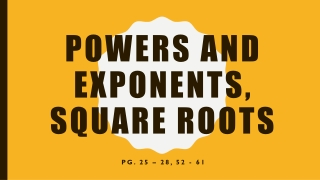DownloadDownload PresentationPowers and Exponents, Square Roots

# Powers and Exponents, Square Roots

Download Presentation## Powers and Exponents, Square Roots

- - - - - - - - - - - - - - - - - - - - - - - - - - - E N D - - - - - - - - - - - - - - - - - - - - - - - - - - -
##### Presentation Transcript

1. Powers and Exponents, Square Roots Pg. 25 – 28, 52 - 61

2. Definitions • FACTORS – Two or more numbers multiplied together to form a product • 5 • 7 = 35 • 5 and 7 are the factors • 35 is the product • When the same factor is used, you can use an exponent. • 3 • 3 = 9, or • 32 = 9 • 3 is the BASE and 2 is the EXPONENT

3. Powers • Numbers expressed by using exponents are called POWERS. • 53 is “ 5 to the third power or 5 cubed. • 53 = 5 • 5 • 5 • 125 • 34 is “3 raised to the fourth power” • 34 = 3 • 3 • 3 • 3 • 81 • 42 is “4 raised to the second power or 4 squared” • 42 = 4 • 4 • 16

4. Write each power as a product of the same factor • 62 • 6 • 6 • 74 • 7 • 7 • 7 • 7 • 26 • 2 • 2 • 2 • 2 • 2 • 2

5. More Definitions • You can EVALUATE, or find the value of, powers by multiplying the factors. • Numbers written without exponents are in STANDARD FORM. • Evaluate 63 • 6 • 6 • 6 • 36 • 6 • 216 • Numbers written with exponents are in EXPONENTIAL FORM. • 9 • 9 • 9 •9 • 94

6. Squares and Square Roots • The product of a number and itself is the SQUARE of that number. • 2 • 2 = 22 = 4 • Numbers like 9, 25 and 81 are called PERFECT SQUARES. • 32 = 9 • 52 = 25 • 92 = 81 • The SQUARE ROOT of a number is one of its two equal factors

7. Estimate square roots • 22 = 4, 32 = 9, 42 = 16, 52 = 25, 62 = 36, 72 = 49, 82 = 64, 92 = 81, 102 = 100 • Estimate the • is not a perfect square. 60 lies between 49 (72) and 64 (82). • Since 60 is closest to 64, the best whole number estimate is 8 • Estimate the • 17 is not a perfect square. It lies between 16 (42) and 25 (52). • Since 17 is closest to 16, the best whole number estimate is 4. • Any number that is not a perfect square has a square root that is an IRRATIONAL NUMBER.